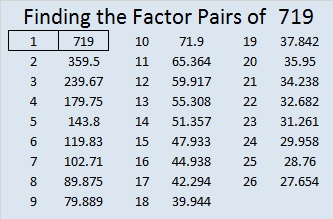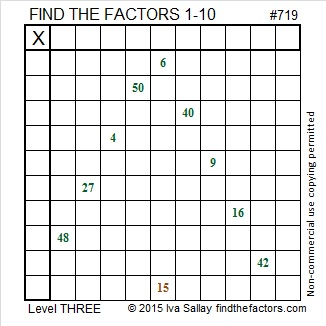# 719 and Level 3

• 719 is a prime number.
• Prime factorization: 719 is prime.
• The exponent of prime number 719 is 1. Adding 1 to that exponent we get (1 + 1) = 2. Therefore 719 has exactly 2 factors.
• Factors of 719: 1, 719
• Factor pairs: 719 = 1 x 719
• 719 has no square factors that allow its square root to be simplified. √719 ≈ 26.814175.

How do we know that 719 is a prime number? If 719 were not a prime number, then it would be divisible by at least one prime number less than or equal to √719 ≈ 26.8. Since 719 cannot be divided evenly by 2, 3, 5, 7, 11, 13, 17, 19, or 23, we know that 719 is a prime number.Here is today’s puzzle:Print the puzzles or type the solution on this excel file: 10 Factors 2015-12-21

—————————————————————————————

Here’s a little more about the number 719:

719 is the sum of the prime numbers from 89 to 113. Can you name those seven prime numbers?

719 is also a palindrome in two other bases:

• 878 BASE 9; note that 8(81) + 7(9) + 8(1) = 719
• 434 BASE 13; note that 4(169) + 3(13) + 4(1) = 719

—————————————————————————————

A Logical Approach to solve a FIND THE FACTORS puzzle: Find the column or row with two clues and find their common factor. (None of the factors are greater than 10.)  Write the corresponding factors in the factor column (1st column) and factor row (top row).  Because this is a level three puzzle, you have now written a factor at the top of the factor column. Continue to work from the top of the factor column to the bottom, finding factors and filling in the factor column and the factor row one cell at a time as you go.